# FOUNDATION Physics Force and Laws of Motion

## Force and Laws of Motion Synopsis

Synopsis

Types of Forces

• We observe various kinds of forces being applied in our daily activities.
• A force is a push or pull on an object.
• It causes a change in the state of rest or motion of an object.
• Its SI unit is newton (N). It is also written as kg.m.s-2.

Balanced and Unbalanced Forces

• If two or more forces act on an object and they do not change the state of rest or motion of the object, then such forces are called balanced forces.
• If two opposite forces are acting on a book placed on a table, then the book will remain at rest if the forces are of equal magnitude.
• Balanced forces cannot change the state of rest or motion of an object. They can however change the shape of the object.
o Example: Pressing a ball changes the shape of the ball, but it does not change its state of rest or motion.
• If two or more forces act on an object and their resultant is not zero, then such forces are called unbalanced forces.
• If two opposite forces are acting on a book placed on a table, then the book will start moving if the forces are of unequal magnitude.
• If the speed or direction of an object changes, then it is due to an unbalanced force acting on the object.
• Thus, force can be defined as an agent, which can produce acceleration in a body on which it acts, or produce a change in its size or shape, or both.
Forces are classified as follows: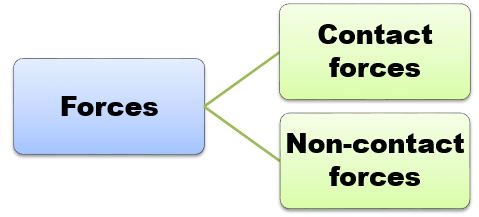• The forces which act on bodies when they are in physical contact are called contact forces.
• When a body moves over a rough surface, a force acts on the body in a direction opposite to the motion of the body along the surface of contact. This is called the frictional force or the force of friction.
• When a person moves towards the right on a road, the force of friction acts on him towards the left. This force resists his motion on the road.
• When a body is placed on a surface, the body exerts a force equal to its weight in the downward direction on the surface. However, the body does not move (or fall) because the surface exerts an equal and opposite force on it, which is called the normal reaction force.
• When a body is suspended by a string, the body pulls the string vertically downwards due to its weight. In its stretched condition, the string pulls the body upwards by a force which balances the weight of the body. This force developed in the string is called the tension force T.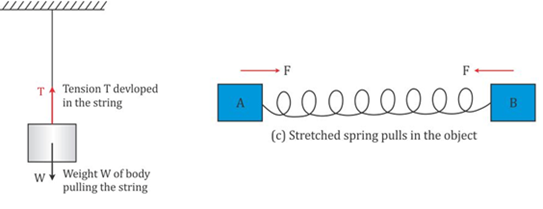• The spring has a tendency to return to its original form. Similarly, when one end of a spring is kept fixed, the spring is found to exert a force at its other end which is directly proportional to the displacement, and the force exerted is in a direction opposite to the direction of displacement. This force is called the restoring force.
• When two bodies collide, they push each other. As a result, equal and opposite forces act on each body.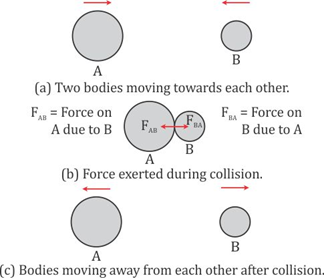• The forces experienced by bodies even without being physically touched are called non-contact forces or forces at a distance.
• In the Universe, each particle attracts another particle because of its mass. This force of attraction between the particles is called the gravitational force.
• The force on a body due to the Earth’s attraction is called the force of gravity. It causes the movement of the body towards the Earth, i.e. downwards, if the body is free to move. The body also attracts the Earth by an equal amount of force, but no motion is caused in the Earth because of its huge mass.
• Two like charges repel, while two unlike charges attract each other. The force between the charges is called the electrostatic force.
• Two like magnetic poles repel each other, while two unlike magnetic poles attract each other. The force between the magnetic poles is called the magnetic force.

Newton’s Laws of Motion

• Newton’s first law: If a body is in a state of rest, then it will remain in that state, and if the body is in a state of motion, then it will continue moving with the same velocity in the same direction unless an external force is applied on it.
• The property of an object by virtue of which it neither changes its state nor tends to change the state is called inertia.
• force is that external cause which tends to change the state of rest or the state of motion of an object.

Mass and inertia

• Mass is a measure of inertia of the body. Greater the mass, greater is the inertia, and thus, more is the resistance to the change in the state of rest or motion.
• The tendency of a body to remain in its state of rest or of uniform motion along a straight line is called inertia. It is due to inertia that an external, unbalanced force must be exerted on the body to change its state of rest or of uniform motion.

Types of inertia

1. Inertia of rest: If a body is at rest, then it will continue to remain at rest unless an external force is applied.
Example: When a bus starts suddenly, the passengers are thrown backwards. This happens because the body tends to stay at rest even after the vehicle has started moving.
2. Inertia of motion: If a body is in a state of motion, then it will continue to move at the same speed in the same direction unless an external force is applied to change its state.
Example: Your bicycle continues to move forward for some time even after you stop pedalling.
This is due to the inertia of motion of the bicycle.
3. Inertia of direction: It is the inability of a body to change its direction of motion along a straight line.
Example: A person, sitting in a moving car will be pushed towards the left, when the car turns suddenly to the right.

Momentum

• The force required to stop a moving body is directly proportional to the mass and velocity of the body.
• Thus, the quantity of motion in a body depends on the mass and velocity of the body. This quantity is termed momentum.
o If a motorcycle travelling with a high speed collides with an object, then the damage is more than that caused by the collision of a slow-moving truck.
• The momentum p of a body is defined as the product of mass m and velocity v of the body.
p = m × v
• If a body is at rest, then its momentum will be zero.
• Momentum has both magnitude as well as direction; hence, it is a vector quantity.
• The SI unit of momentum is kg.m/s.

• Newton’s second law: The rate of change of momentum of a body is directly proportional to the applied force and occurs in the direction in which the force acts.• Consider a body of mass m moving with initial velocity u. Its initial momentum will be mu. When a force F acts on the body for time t, its velocity becomes v. Thus, final momentum is mv. Thus, Newton’s second law is written asIn SI units, k = 1. Thus, we have
F = ma
• Thus, Newton’s second law of motion provides a method to measure the force on a body as a product of its mass and acceleration.
• We can deduce the statement of the first law from the second law.

F = maFT = mv - mu
• Thus, if F = 0, then v = u.
• That is, if the external force is zero, then the body continues to move uniformly throughout its motion.

Applications of Newton’s Second Law of Motion

• Catching a cricket ball: When a fielder catches a cricket ball, he drags his hands backwards in order to increase the time taken by the ball to reduce its momentum to zero. This reduces the rate of change of momentum, thereby decreasing the force exerted on the hands by the ball.
• Seat belts in a car: When brakes are suddenly applied or a car crashes with an object, the seat belts increase the time taken to move forward, thereby decreasing the rate of change of momentum. Thus, the force of impact is reduced and fatal accidents can be prevented.
• Newton’s Third law of motion:  To every action, there is an equal and opposite reaction.
• When you stand on the ground, you are actually pushing the ground and the ground is pushing you by an equal amount of force.
• When you pull a spring with both hands, you feel an equal force trying to pull the spring back.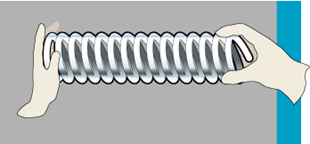• Thus, action and reaction forces are present. However, the action and reaction forces act on two different bodies.
• Examples:
Recoil of a pistol: When a person fires a gun, he gets a push in the backward direction.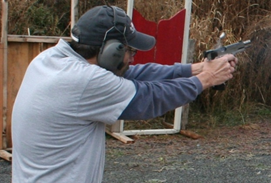oSwimming: While swimming, a person pushes against the water. The water exerts an equal and opposite force on the person enabling forward movement.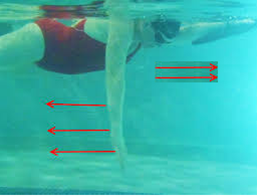oRockets work on the action–reaction forces.
oBoats move in water using the same principle.

Conservation of Momentum

• We know that momentum is the product of mass and velocity.
p = m × v
• Consider a collision between a moving and a stationary object. When the collision occurs, the velocity of the moving object decreases. But at the same time, the velocity of the stationary object increases.
• This increase in momentum of one object is equal to the decrease in momentum of the other. Thus, there is no loss of momentum.
• Hence, the law of conservation of momentum can be stated as, “The sum of momenta of objects before collision is equal to the sum of momenta after collision, provided there is no external force acting on the objects”.
• Consider two objects A and B of masses mA and mB initially moving in a straight line with velocities uA and uB, respectively. Assume that uA > uB. They collide with each other as shown below.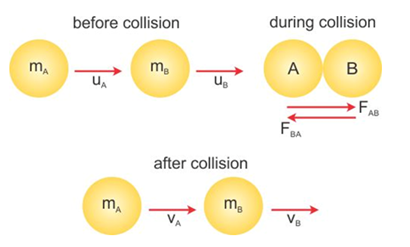• The collision lasts for time t. During this collision, A exerts a force FAB on B and B exerts a force FBA on A.
• Suppose vA and vB are the velocities of A and B after collision. The momentum before and after collision for object A is mAuA and mAvA. Thus, the force FAB is• Similarly, the force FBA for object B on A is• According to the third law of motion, we have  FAB = -FBA
• Thus, we getmA(vA - uA) = -mB(vB - uB)
∴ mAu+ mBuB = mAv+ mBvB
• The total momentum of both objects A and B before collision is equal to the total momentum of both objects after collision. Thus, the momentum is conserved in a collision.

Impulse

• When a sharp knock is given to a door, the moving finger has momentum. Once the door is struck, the momentum of the finger is reduced to zero in a very short interval of time.
As a result, the force imparted on the door is very large in a short interval of time, finger get hurt.
• This large force acting for a short interval of time is called impulsive force. The product of force and time during which the force act is called impulse.
Impulse = force × time
∴ Impulse = mass × acceleration × time = m a × t• Thus, impulse can be defined as change in momentum. Like momentum, impulse is a vector quantity.
• Unit of impulse in S.I. system is N s or kg m s−1 and in C.G.S. system, it is dyne second or g cm s–1.
Examples: -
• A cricket fielder lowers his hands while catching a ball. If the ball is caught without lowering the hands, the fielder will hurt his hands due to a large force. When the ball is caught by moving the hand in the direction of motion of the ball, the duration of the impact increases. As a result, the rate of change of momentum decreases, and thus, the force exerted by the ball on the hand is reduced.
• An athlete taking a long jump or a high jump bends his knees before landing. By doing so, he increases the time of fall. This decreases the rate of change of momentum and this greatly reduces the impact of fall.
• Thus, from the above examples, it is clear that the rate of change of momentum can be increased or decreased, respectively, by decreasing or increasing the time of contact.

Thrust and Pressure

• Thrust is the force acting perpendicularly on an object.
• The effect of thrusts of same magnitude on different areas is different.
• Pressure is the force acting perpendicularly on a unit area of the object.• When we stand on sand, our feet go deep into the sand. However, if we sleep on sand, our body does not go that deep. Although the same force (your weight) acts on the sand, the area on which the force acts is different. The effect of force is larger while standing than while lying down.
• Thus, the same force when acting on a smaller area exerts a larger pressure, and when acting on a larger area exerts a smaller pressure.
• The SI unit of thrust/force is newton (N) and that of pressure is N/m2.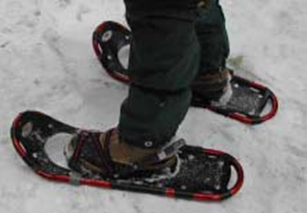Man walking on snow: Pressure is reduced because of large area of shoes.